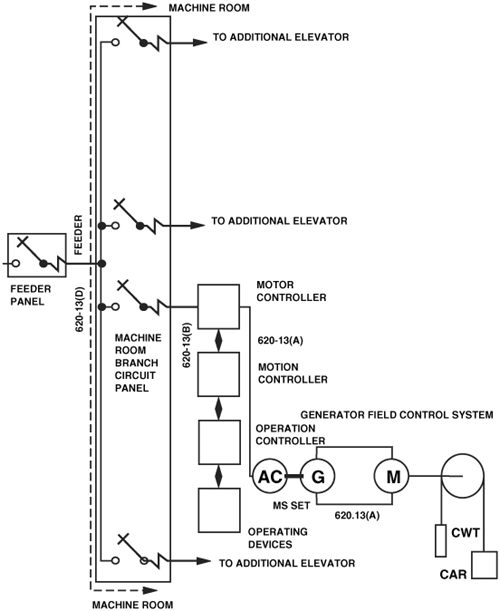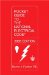# Example No. D9 Feeder Ampacity Determination for Generator Field Control

(See 215.2, 430.24, 430.24 Exception No. 1, 620.13, 620.14, 620.61, and Tables 430.22(E) and 620.14.)

Determine the conductor ampacity for a 460-volt 3-phase, 60-Hz-ac feeder supplying a group of six elevators.

The 460-volt-ac drive motor nameplate rating of the largest MG set for one elevator is 40 horsepower, and 52 amperes, and the remaining elevators, each have a 30-horsepower, 40-ampere, ac drive motor rating for their MG sets. In addition to a motor controller, each elevator has a separate motion/operation controller rated 10 amperes continuous to operate microprocessors, relays, power supplies, and the elevator car door operator. The MG sets are rated continuous.

Conductor Ampacity

Conductor ampacity is determined as follows:

(a) Per 620.13(D) and 620.61(B)(1) use Table 430.22(E), for intermittent duty (elevators). For intermittent duty using a continuous rated motor, the percentage of nameplate current rating to be used is 140 percent.

(b) For the 30-horsepower ac drive motors, 140% x 40 amps = 56 amperes.

(c) For the 40-horsepower ac drive motors, 140% x 52 amps = 73 amperes.

(d) The total conductor ampacity is the sum of all motor currents (1 motor x 73 amperes) + (5 motors x 56 amperes) = 353 amperes.

(e) Per 620.14 and Table 620.14, the conductor (feeder) ampacity shall be permitted to be reduced by the use of a demand factor. Constant loads are not included. (See 620.14, FPN). For six elevators, the demand factor is 0.79. Therefore feeder diverse ampacity is, 0.79 x 353 amperes = 279 amperes.

(f) Per 430.24 and 215.3, controller continuous current is 125% x 10 amperes = 12.5 amperes.

(g) The total feeder ampacity is the sum of the diverse current and all the controller continuous current. I total = 279A + (6 elevators x 12.5) = 354 amperes.

(h) This ampacity shall be permitted to be used to select the wire size.

See Figure D9.

Figure D9. Generator field controlPocket Guide to the National Electrical Code(R), 2005 Edition (8th Edition)
ISBN: 0131480014
EAN: 2147483647
Year: 2004
Pages: 120